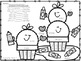# Multi-Digit Multiplication Color-By-Number Back To School ThemedSubject
Resource Type
File Type

PDF

(1 MB|7 pages)
Product Rating
4.0
(5 Ratings)
Standards
• Product Description
• StandardsNEW
Do your students need a little extra practice with multiplication facts? Here is a great way to have a little fun while practice multi-digit multiplication facts. Great for early finishers, sub days, RTI, morning work, indoor recess, and much more!

Included in this multiplication packet:
- 2 digit by 1 digit (2)
- 2 digit by 2 digit
- 3 digit by 1 digit
- 3 digit by 2 digit
- 3 digit by 3 digit
- A mix of all

4.NBT.5 - Multiply a whole number of up to four digits by a one-digit whole number, and multiply two two-digit numbers, using strategies based on place value and the properties of operations. Illustrate and explain the calculation by using equations, rectangular arrays, and/or area models.

5.NBT.5 - Fluently multiply multi-digit whole numbers using the standard algorithm.
Fluently multiply multi-digit whole numbers using the standard algorithm.
Multiply a whole number of up to four digits by a one-digit whole number, and multiply two two-digit numbers, using strategies based on place value and the properties of operations. Illustrate and explain the calculation by using equations, rectangular arrays, and/or area models.
Fluently add and subtract multi-digit whole numbers using the standard algorithm.
Total Pages
7 pages
N/A
Teaching Duration
N/A
Report this Resource to TpT
Reported resources will be reviewed by our team. Report this resource to let us know if this resource violates TpT’s content guidelines.